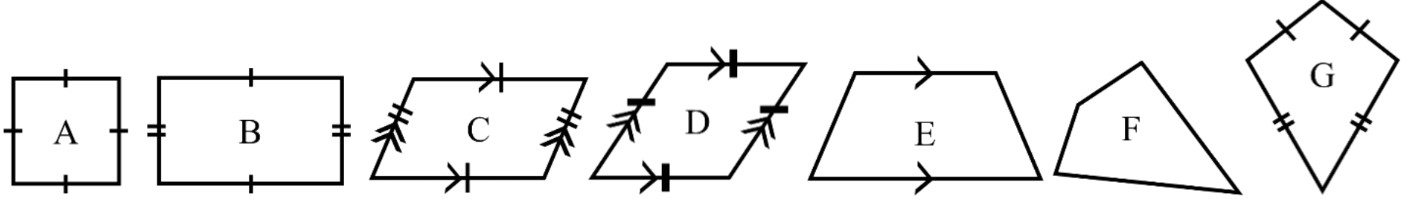#### All in One Place

Everything you need for JC, LC, and college level maths and science classes.#### Learn with Ease

We’ve mastered the national curriculum so that you can revise with confidence.#### Instant Help

24/7 access to the best tips, walkthroughs, and practice exercises available.

0/6
##### Intros
###### Lessons
1. Introduction to Classifying Quadrilaterals:
2. What is a quadrilateral and what geometry concepts are we going to use to classify different types?
3. Classifying rectangles and squares
4. Classifying parallelograms and rhombuses
5. Classifying trapezoids
6. Classifying kites
7. A review on the 6 different classifications of quadrilaterals
0/21
##### Examples
###### Lessons
1. Naming quadrilateral shapes
Classify the quadrilateral. Think about its sides and angles.
1.2.3.4.5.6.2. Classifying quadrilateral types
Answer with the letter on each quadrilateral shape1. Which shapes are quadrilaterals?
2. Which shapes are parallelograms?
3. Which shapes are rectangles?
4. Which shapes have all 4 sides congruent?
5. Which shapes do not have right angles?
6. Which shapes have exactly 2 pairs of congruent sides?
3. Angles of quadrilaterals
Find the missing angle(s) and classify the quadrilateral.
1.2.3.4.4. Classifying quadrilaterals - word problems
1. I have 2 pairs of parallel & congruent sides. If I do not have right angles, what am I?
2. I have 4 congruent sides and these sides make up 2 pairs of parallel sides. If I only have right angles, what am I?
3. I have a total angle sum of 360° and only 1 pair of parallel sides. What am I?
4. I am to a parallelogram what a square is to a rectangle. What am I?
5. I have 2 pairs of adjacent congruent sides and 1 pair of equal opposite angles. What am I?
0%
##### Practice
###### Free to Join!
StudyPug is a learning help platform covering math and science from grade 4 all the way to second year university. Our video tutorials, unlimited practice problems, and step-by-step explanations provide you or your child with all the help you need to master concepts. On top of that, it's fun - with achievements, customizable avatars, and awards to keep you motivated.
• #### Easily See Your ProgressWe track the progress you've made on a topic so you know what you've done. From the course view you can easily see what topics have what and the progress you've made on them. Fill the rings to completely master that section or mouse over the icon to see more details.
• #### Make Use of Our Learning Aids###### Practice Accuracy

Get quick access to the topic you're currently learning.

See how well your practice sessions are going over time.

Stay on track with our daily recommendations.

• #### Earn Achievements as You LearnMake the most of your time as you use StudyPug to help you achieve your goals. Earn fun little badges the more you watch, practice, and use our service.
• #### Create and Customize Your AvatarPlay with our fun little avatar builder to create and customize your own avatar on StudyPug. Choose your face, eye colour, hair colour and style, and background. Unlock more options the more you use StudyPug.
###### Topic Notes

In this lesson, we will learn:

• A quadrilateral is a polygon with 4 sides
• We can look at the properties of a quadrilateral (pairs of parallel sides, pairs of congruent sides, types of angles) to classify into 6 different types
• The special types of quadrilaterals are: rectangle, square, parallelogram, rhombus, trapezoid, and kite

Notes:

• A quadrilateral is a 2D shape with 4 straight sides (a 4-sided polygon)
• A quadrilateral also has 4 vertices (corners)
• Each corner contains an angle; the total angle sum in any quadrilateral is 360°

• There are special quadrilaterals with specific definitions and names
• There will be 6 types of quadrilaterals in this lesson: Rectangle, Square, Parallelogram, Rhombus, Trapezoid, and Kite
• A quadrilateral can fit the definition of more than one of these special types

• Rectangles and squares:
• Quadrilaterals with 4 right angles (all angles are 90° )
• A rectangle has 2 pairs of opposite and equal sides (congruent)
• A square is a special type of rectangle where all 4 sides are equal (congruent)
• All squares are rectangles, but not all rectangles are squares
• Rectangles and squares can also be considered as parallelograms, just with right angles

• Parallelograms and rhombuses:
• Quadrilaterals with 2 pairs of parallel sides and 2 pairs of opposite equal angles
• A parallelogram has 2 pairs of congruent sides (equal length)
• A rhombus has all 4 sides congruent (all 4 sides equal length)
• All rhombuses are parallelograms; not all parallelograms are rhombuses

• Trapezoids:
• Quadrilaterals with only 1 pair of parallel sides

• Kites:
• Quadrilaterals with 2 adjacent congruent sides (no parallel sides)
• There is 1 pair of opposite equal angles across the line of symmetry

• Summary of quadrilateral types and properties (sides and angles):